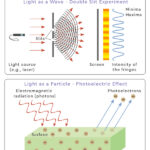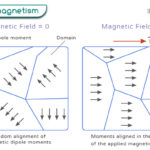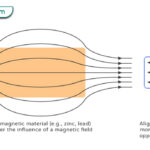Home / Physics / Friction

# Friction

## What is Friction

Friction or frictional force is defined as the force that resists an object’s motion on a surface. The object can either be stationary or in motion relative to the surface. Friction occurs where the object is in contact with the surface. In other words, it takes place between two surfaces, and hence, is a contact force.

Surfaces are not perfectly smooth. They consist of bumps and irregularities. When two surfaces come in contact, these bumps adhere and interlock, making it difficult for the surfaces to move. Because of this resistance towards motion, friction is considered as a force.

The frictional force is non-conservative. When an object moves from one position to another against friction, energy is not conserved as some of it is lost through heat.

## Types of Friction

There are two main types of friction.

### 1. Static Friction

Static friction arises when the object is stationary relative to the surface. If a force tries to displace it, the friction is strong enough to hold the object in its position. The static friction increases with the applied force until it reaches a maximum value. Once the applied force exceeds this value, the object starts to move, and static friction ceases to exist.

#### Examples

Examples of static friction include the force that resists

• a person from slipping while walking and running.
• a heavy object from moving while pushing or pulling.

### 2. Kinetic Friction

Kinetic friction arises when the object moves relative to the surface after the applied force overcomes the static friction. Kinetic friction can be of two types. If the object is sliding over the surface, then it experiences sliding friction. If the object rolls over the surface, then it is called rolling friction.

#### Examples

Examples of kinetic friction include the force that is responsible for

• a car to skid
• a skier to come to a stop

Aside, there is another type of friction.

### 3. Fluid Friction

Fluid friction arises when an object moves through a fluid. It can also occur between two layers of fluid that move relative to one another. The fluid can be gas (e.g., air) or liquid (e.g., water). In some fluids like oil and water, the friction depends on the fluid’s viscosity.

#### Examples

Examples of fluid friction include the force experienced by

• a swimmer while swimming in a pool
• a ship sailing in the ocean

## Characteristics of Frictional Force

Here are some facts and properties of the frictional force.

• Opposes the relative motion between the object and surface
• Reduces the velocity of the object and causes it to stop
• Performs negative work
• Generates heat at the point of contact
• Low for smooth surfaces and high for rough surfaces (Effect of surface roughness has been a study for many researchers over the years)

## Laws of Friction

The frictional force obeys a few laws.

• Proportional to the normal load or normal force
• Independent of the area of contact between the surfaces
• Depends on the nature of the surfaces in contact
• Kinetic friction is independent of the velocity of the moving object

## How to Find Frictional Force

The frictional force can be found by applying the friction laws and Newton’s laws of motion.

### Force of Friction Equation

According to the laws of friction, the frictional force F is proportional to the normal force or normal load FN. The effect of the normal force is such that friction increases with it. This law can be represented in the form of an equation.

F FN

Or, F = μFN

Symbol

Unit of frictional force: Newton or N

Dimensions of frictional force: MLT-2

This equation gives the magnitude of the frictional force. Its direction is opposite to the applied force. The constant of proportionality μ is called the friction coefficient or coefficient of friction (COF). Unlike other proportionality constant, it does take a constant value and has no unit. Its value depends upon the two surfaces in contact.

#### Frictional Force on a Horizontal Surface

Suppose a block of mass m is lying on a table. A force is applied to the block, which tries to displace it. However, due to friction, the block remains unmoved. From Newton’s third law of motion, the force applied by the block on the table is equal to the force applied by the table on the block, which is the static frictional force FS.

FS = μSFN

Where,

μS is calledthe coefficient of static friction.

Again, using Newton’s third law, the normal force exerted by the table on the block equals the block’s weight. Weight is defined as mass m multiplied by the acceleration due to gravity g.

FN = mg

Therefore,

FS = μSmg

Now, suppose that the applied force FA is large enough to displace the object, which moves with an acceleration a. According to Newton’s second law, the net force with which it is moving is

ma = FA – FK

Where FK is the kinetic friction force, which, according to friction laws, is given by,

FK = μKFN

Or, FK = μKmg

Where,

μK is calledthe coefficient of kinetic friction, which is less than the coefficient of static friction.

The force on the block is,

ma = FAμKmg

Therefore, the acceleration of the block is,

a = FA/m – μKg

The work done by the frictional force is the force multiplied by the displacement d.

W = FKd

#### Frictional Force on Inclined Plane

Suppose the block is motionless on an inclined plane that makes an angle θ with the horizontal.  Its weight mg can be resolved into two components – one parallel to the surface and the other perpendicular to the surface.

Perpendicular component: mg cos θ

From Newton’s third law,

FN = mg cos θ

From laws of friction,

FS = μSmg cos θ

Parallel component: mg sin θ

Suppose the inclination angle is raised, and the block starts to move with acceleration a down the wedge.

Again, from laws of friction,

FK = μKmg cos θ

And, according to Newton’s second law of motion,

ma = mg sin θ – FK

Or, ma = mg sin θ – μKmg cos θ

Or, μK = (g sin θ – a)/g cos θ

If the acceleration is zero, then,

μs = sin θ/cos θ = tan θ

The above equation can be used to calculate the friction coefficients.

## Factors Affecting Frictional Force

Here are the factors that affect the frictional force.

• Nature of the surface: Smooth surfaces have low friction, whereas rough surfaces have high friction. A rough surface can be made smooth by polishing to reduce friction.
• Weight of the object: From the laws of friction, it is quite clear that the more massive the object, the higher is the force of friction.

• Makes motion on a surface possible (e.g., walking, running, driving, skating, and writing)
• Prevents slipping and sliding (e.g., a ladder can stay on the wall, and objects can pile up on top of one another)
• Allows vehicles to stop using brakes
• Keeps hands warm in winter when rubbed against each other
• Burns asteroids when they enter Earth’s atmosphere

• Opposes and slows down the motion
• Requires extra energy to push and pull heavy objects
• Makes object wear and tear easily (e.g., an eraser wears due to rubbing, and machine parts wearing due to regular use)
• Generates unnecessary heat (e.g., brakes of a car get heated due to continuous pumping of the brake pedal, and the wheels get heated and give off smoke when it rotates in the snow without the vehicle moving forward)

## FAQs

Q.1. What is the apparatus used to measure friction force?

Ans. The apparatus used to measure friction force is called a tribometer.

Article was last reviewed on Friday, February 17, 2023

### Related articlesWave-Particle DualityPhotoelectric EffectFerromagnetismDiamagnetism